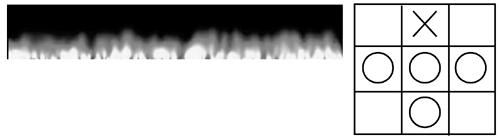# Texture Mapping

Many texture mapping tricks are possible with shaders. Again, most of these techniques can be resolved at the vertex shader level or in a fragment program. Vertex shaders can change the mapping coordinates of a vertex, and thus full texture mapping can be implemented this way. Fragment shaders are a great place to compute procedural textures, which are computed not as texture coordinates, but actually shade each fragment with a mathematical function.

In this next example, I will compute a vertex shader that implements texture animation on the GPU. The idea is really straightforward. We have a global texture map that encodes several frames of a looping animation. Think of a flame with n subtextures encoded into one. We want to pass a timer to the shader so it automatically generates texture coordinates for the frame we are in. Here is the source code for this example:

` void flames       (float3 position: POSITION, float4 color: COLOR, float2 texcoord: TEXCOORD0, out float4 oPosition: POSITION, out float2 oTexCoord: TEXCOORD0, out float3 oColor: COLOR, uniform float timevalue, uniform float speed, uniform float4x4 modelviewProj) { int frame=abs(speed/timevalue); frame=frame%16; int row=frame%4; int col=frame/4; float xCoord=row*0.25+texcoord.x*0.25; float yCoord=col*0.25+texcoord.y*0.25; oPosition=mul(modelviewProj, position); oTexCoord=float2(xCoord, yCoord); oColor=color; } `

Notice how the shader's source computes the frame we are at, and then translates that into row and column numbers to index our texture map, generating texturing coordinates for the flame.

The most interesting texture effects lie on the fragment program side. Having control on a per-texel basis gives us the flexibility we need to create unique textures. As an example, here is a fire generator by Damian Trebilco.

` void fire (float4 Position : POSITION, float3 Tex0     : TEXCOORD0, float3 Tex1     : TEXCOORD1, float3 Tex2     : TEXCOORD2, float3 Tex3     : TEXCOORD3, out float4 oColor     : COLOR, uniform sampler2D FireMap,                   uniform sampler1D LookupMap,                   uniform float3 fireVar) { float4 currentIndex; float index; //Sample the four texture positions (The texture width is in the z component) currentIndex =f4tex2Dproj(FireMap, Tex0); currentIndex +=f4tex2Dproj(FireMap, Tex1); currentIndex +=f4tex2Dproj(FireMap, Tex2); currentIndex +=f4tex2Dproj(FireMap, Tex3); //Get the average index=currentIndex.a *0.25; //Decrease by a large amount for all initial values if(index > fireVar.x)    {    index = index - fireVar.y;    } //When the limit is reached, drop off slower else    {    index = index - fireVar.z;    } //Clamping the index is not really necessary unless a floating point target is used clamp(index,0.0,1.0); //Look up the new color in the palette oColor.xyz =h3tex1D(LookupMap,index); oColor.w   =index; } `

This program requires some explanation. Fire was a popular effect in the demo scene in the late 1980s. It was computed by painting white pixels at the bottom of the screen, and then using a cellular automata-style routine to propagate the fire to the pixels above it. For each frame, each pixel was scanned, and its new color value was the average of the color values of the pixels located below it, as shown in Figure 21.5.

##### Figure 21.5. Cellular fire. Left: End result. Right: Explanation. To compute a fire sample at the position X in frame N+1, we average the values at pixels marked O at frame N.Computing fire on a shader is very similar to this approach. We need the fragment program to receive the pixel to be shaded and the values of the pixels to be taken into consideration. To do so, we use a sampler, which is a Cg object that allows us to retrieve values from textures. The first four lines of the shader retrieve the indices of these four pixels. We then average these values, which causes the bleeding and vertical propagation fire effects are known for. Then, the if clause is added only to regulate the speed at which flames fade away: faster if above a certain threshold and slower afterward. However, fire does not work on RGB space, but instead works on index space, as in a palettized environment. Thus, we need to do a table lookup using a 1D sampler to retrieve the RGB values associated with the index we have computed for this point.

This effect is a very good showcase of how procedural textures are generated.Core Techniques and Algorithms in Game Programming2003
ISBN: N/A
EAN: N/A
Year: 2004
Pages: 261